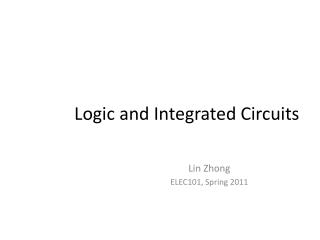DownloadDownload PresentationLogic and Integrated Circuits

# Logic and Integrated Circuits

Télécharger la présentation## Logic and Integrated Circuits

- - - - - - - - - - - - - - - - - - - - - - - - - - - E N D - - - - - - - - - - - - - - - - - - - - - - - - - - -
##### Presentation Transcript

1. Logic and Integrated Circuits Lin Zhong ELEC101, Spring 2011

2. Key concepts • Binary numeral system • Boolean logic • Logic gates • Functional completeness • CMOS gates • Integrated circuits

3. Binary computing • Modern computing are based on binary states • Two values: HIGH vs. LOW, 1 vs. 0, true vs. false • Why • Easy to implement • Robust against interference, noise,

4. Computing with binary states • Binary numeral system • Represent numeric values using two values: 0 and 1 • The more “natural” numeral system is decimal • 0, 1, 2, 3, 4, 5, 6, 7, 8, 9 • One to one mapping between the two systems

5. Recall the single-input computer • Inverter Out In

6. How about a two-to-one computer Binary “states” for input and output: HIGH or LOW (1 or 0) A Out B How many different computers are there?

7. How about a two-to-one computer Binary “states” for input and output: HIGH or LOW (1 or 0) A Out B Useless ones: Out always 1; Out always 0; Out=A; Out=B Inverter Out= Invert (A); Out= Invert (B) Useful ones:????

8. Three basic logic operations • Inversion (NOT): Out = ¬ In • AND: Out = A Λ B • OR: Out = A V B

9. More gates • NAND • NOR • XOR • A XOR B = [A Λ (¬B)] V [(¬A) Λ B]

10. How about a two-to-one computer Binary “states” for input and output: HIGH or LOW (1 or 0) A Out B Useless ones: Out = 0; Out =1; Out =A; Out=B; Inverters: Out= ¬A; Out= ¬B; Useful ones: Out = A Λ B(AND), Out= A V B(OR), Out= A XOR B Out = ¬(A Λ B) (NAND), Out= ¬ (A V B) (NOR), Out = ¬ ( A XOR B) A Λ (¬B); (¬A) Λ B; A V (¬B); (¬A)V B;

11. Functional completeness • NOT, AND and OR can be used to build ANY Boolean function • Functionally complete • Can you prove the following? • NOR is functionally complete • NAND is functionally complete

12. CMOS gates implementation • NOT • AND • OR

13. CMOS gates: NOR Vdd A B Out Gnd

14. Lab: NAND gate

15. Adder A B A, B, and Sum are states that take value from 0 to 9 Carry-out is a state that take value from 0 to 1 Carry-out Sum

16. The simplest adder A, B, and Sum are states that take value from 0 to 1 Carry-out is a state that take value from 0 to 1 A B Carry-out Truth table Sum

17. The simplest adder (Contd.) A, B, and Sum are states that take value from 0 to 1 Carry-out is a state that take value from 0 to 1 A B Carry-out Truth table Sum Sum= A XOR B Carry-out = A AND B

18. How many transistors do you need? Carry-out Sum= A XOR B Carry-out = A AND B Sum

19. AND

20. XOR • Sum= A XOR B = [A Λ (¬B)] V [(¬A) Λ B] • = [A AND (NOT B)] OR [(NOT A) AND B] • =[A NAND (NOT B)] NAND [(NOT A) NAND B] • = [(A AND B) NOR (A NOR B)]

21. XOR (Contd.) Out

22. XOR (Contd.) Vdd Out

23. Computing with binary states (Contd.) • Boolean logic • Variables are binary (0 or 1) • Three operations on binary variables • Inversion (¬), AND (Λ), and OR (V) • Five axioms George Boole 1815-1864

24. Integrated Circuit Shared Nobel Prize in Physics 2000

25. Photolithography

26. 1969

27. Ivan Sutherland won Turing Award in 1988 for his Ph.D. work in 1963 http://www.cl.cam.ac.uk/techreports/UCAM-CL-TR-574.pdf

28. “Programmable” integrated circuit A B Carry-out Sum

29. “Programmable” integrated circuit A B Control Output

30. “Programmable” integrated circuit Storage A B Control Output

31. “Programmable” integrated circuit Storage A B Control Output

32. “Programmable” integrated circuit Storage A B Instruction in machine code 0110100010101 Control Output

33. “Programmable” integrated circuit Storage A B Control Default start instruction Output

34. Computing vs. human performance Sources: intel.com and factmonster.com

35. Computing vs. humanity Source: Intel.com and dol.gov

36. Computing: 60 years ago Wiring ENIAC with a new program http://ftp.arl.army.mil/ftp/historic-computers/png/eniac4.png